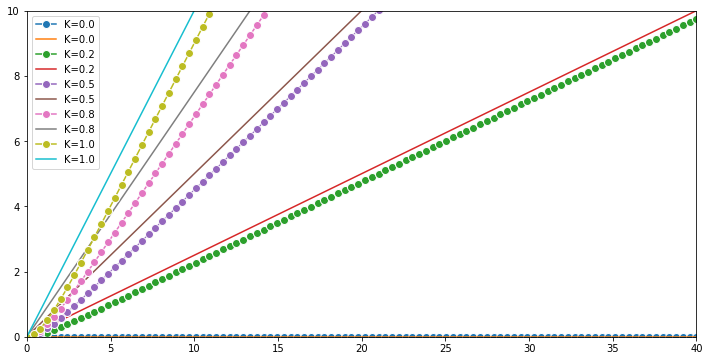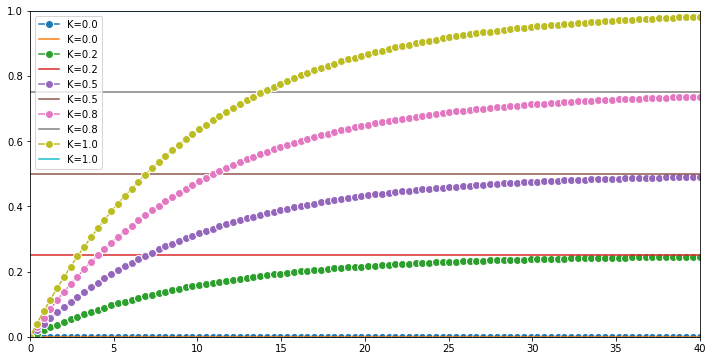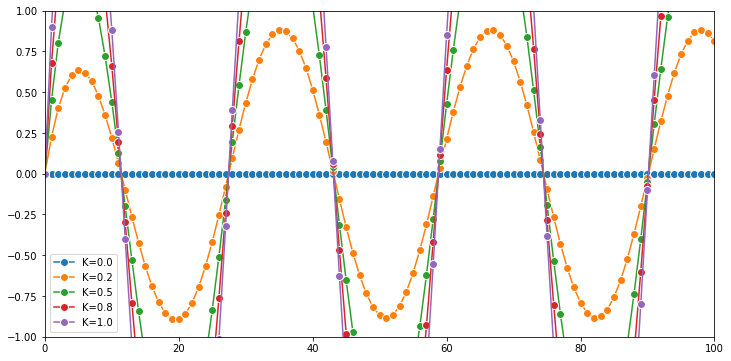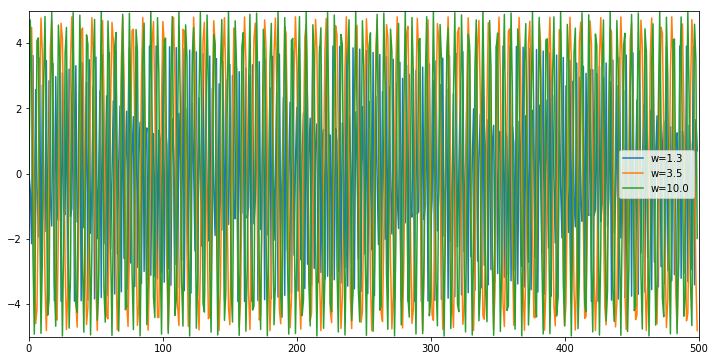In :
import numpy as np
import scipy.integrate as spi
import matplotlib.pyplot as plt
%matplotlib inline

In :
A = 1.  # Amplitude
K = 1.  # Static Sensitivity b_0 / a_0
tau = 1  # Time Constant a_1 / a_0

In :
# The initial voltage is 0.
v0 = 0

In :
def g(v, t0, K, A, tau):
# We compute the first derivative v' of v.
vdot = -v/tau + K*(A/tau)*t0
# We return v'=vdot.
return vdot

In :
fig2, ax = plt.subplots(1, 1, figsize=(12, 6))

# We want to evaluate the system on 100 linearly
# spaced times between t=0 and t=20.
t = np.linspace(0., 40., 100)

# We simulate the system for different values of K.
for K in np.linspace(0., 1., 5):
# We simulate the system and evaluate $v$ on the
# given times.
v = spi.odeint(g, v0, t, args=(K,A,tau))
inp = A*K*t
# We plot the particle's trajectory.
ax.plot(t, v, 'o-', mew=1, ms=8,
mec='w', label=f'K={K:.1f}')
ax.plot(t, inp, '-', mew=1, ms=8,
mec='w', label=f'K={K:.1f}')
ax.legend()
ax.set_xlim(0, 40)
ax.set_ylim(0, 10)

Out:
(0, 10)In :
A = 1.  # Amplitude
K = 1.  # Static Sensitivity b_0 / a_0
tau = 10  # Time Constant a_1 / a_0
# The initial voltage is 0.
v0 = 0

In :
def h(v, t0, K, A, tau):
# We compute the first derivative v' of v.
vdot = -v/tau + K*(A/tau)
# We return v'=vdot.
return vdot

In :
fig2, ax = plt.subplots(1, 1, figsize=(12, 6))

# We want to evaluate the system on 100 linearly
# spaced times between t=0 and t=20.
t = np.linspace(0., 40., 100)
inp_t = np.ones(100)
# We simulate the system for different values of K.
for K in np.linspace(0., 1., 5):
# We simulate the system and evaluate $v$ on the
# given times.
v = spi.odeint(h, v0, t, args=(K,A,tau))
inp = A*K*inp_t
# We plot the particle's trajectory.
ax.plot(t, v, 'o-', mew=1, ms=8,
mec='w', label=f'K={K:.1f}')
ax.plot(t, inp, '-', mew=1, ms=8,
mec='w', label=f'K={K:.1f}')
ax.legend()
ax.set_xlim(0, 40)
ax.set_ylim(0, 1)

Out:
(0, 1)In :
A = 1.  # Amplitude
K = 1.  # Static Sensitivity b_0 / a_0
tau = 1  # Time Constant a_1 / a_0
w = 1 # Frequency of driving force

In :
# The initial voltage is 0.
v0 = 0

In :
def f(v, t0, K, A, tau):
# We compute the first derivative v' of v.
vdot = -v/tau + 5*K*(A/tau)*np.cos(w*t0)
# We return v'=vdot.
return vdot

In :
fig, ax = plt.subplots(1, 1, figsize=(12, 6))

# We want to evaluate the system on 100 linearly
# spaced times between t=0 and t=100.
t = np.linspace(0., 100., 500)

# We simulate the system for different values of K.
for K in np.linspace(0., 1., 5):
# We simulate the system and evaluate $v$ on the
# given times.
v = spi.odeint(f, v0, t, args=(K,A,tau))
# We plot the particle's trajectory.
ax.plot(v, 'o-', mew=1, ms=8,
mec='w', label=f'K={K:.1f}')
ax.legend()
ax.set_xlim(0, 100)
ax.set_ylim(-1, 1)

Out:
(-1, 1)In :
A = 1.  # Amplitude
K = 1.  # Static Sensitivity b_0 / a_0
tau = 1  # Time Constant a_1 / a_0
w = 1 # Frequency of driving force

In :
# The initial voltage is 0.
v0 = 0

In :
fig, ax = plt.subplots(1, 1, figsize=(12, 6))

# We want to evaluate the system on 100 linearly
# spaced times between t=0 and t=100.
t = np.linspace(0., 1000., 500)

# We simulate the system for different values of K.
for w in np.logspace(0.1, 1., 3):
# We simulate the system and evaluate $v$ on the
# given times.
v = spi.odeint(f, v0, t, args=(w,A,tau))
# We plot the particle's trajectory.
ax.plot(v, '-', mew=1, ms=8,
mec='w', label=f'w={w:.1f}')
ax.legend()
ax.set_xlim(0, 500)
ax.set_ylim(-5, 5)

Out:
(-5, 5)In [ ]:


In [ ]: